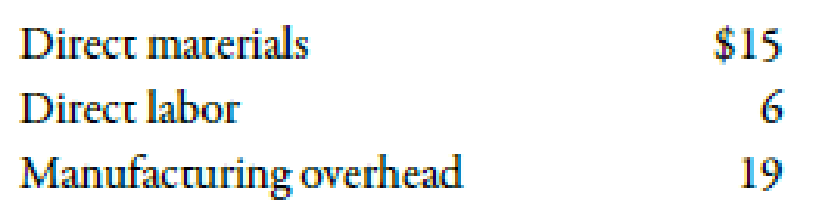Chapter 2, Problem 4MCQ### Managerial Accounting: The Corners...

7th Edition
Maryanne M. Mowen + 2 others
ISBN: 9781337115773

#### Solutions

Chapter
Section### Managerial Accounting: The Corners...

7th Edition
Maryanne M. Mowen + 2 others
ISBN: 9781337115773
Textbook Problem
1 views

# Use the following information for Multiple-Choice Questions 2-3 and 2-4:Wachman Company produces a product with the following per-unit costs:Last year, Wachman produced and sold 2,000 units at a price of $75 each. Total selling and administrative expense was$30,000.2-4    Refer to the information for Wachman Company on the previous page. Total gross margin for last year was a. $40,000. b.$70,000. c. $80,000. d.$88,000. e. $100,000. To determine Calculate the amount of gross margin. Explanation Gross Margin: Gross margin can be defined as the difference between the sales revenue and COGS (cost of goods sold). It refers to the amount of money that the firm is earning over and above the amount of cost of sold units. b. Use the following formula to calculate the gross margin: Gross margin=Sales revenueCOGS Substitute$150,000 for sales revenue and $80,000 for COGS in the above formula. Gross margin=$150,000$80,000=$70,000

The amount of gross margin is $70,000. Therefore, this option is correct. Working Notes: Calculation of sales revenue: Sales revenue=Units produced and sold×Selling price=2,000units×$75=$150,000 Hence, the amount of sales revenue is$150,000

### Still sussing out bartleby?

Check out a sample textbook solution.

See a sample solution

#### The Solution to Your Study Problems

Bartleby provides explanations to thousands of textbook problems written by our experts, many with advanced degrees!

Get Started